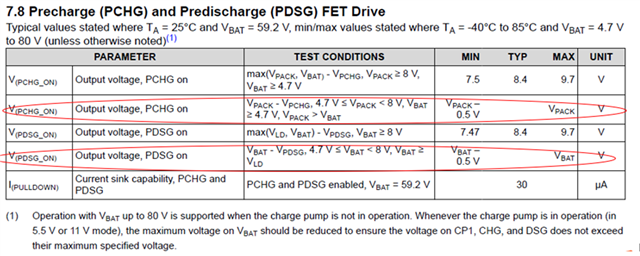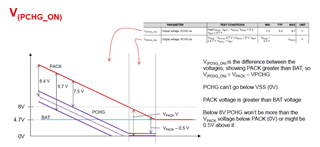If you have a related question, please click the "Ask a related question" button in the top right corner. The newly created question will be automatically linked to this question.

# [FAQ] BQ76952: What is the default output of PDSG/PCHG when pre-discharge and pre-charge is enabled

Part Number: BQ76952

Hi team,

1. What is the output voltage for PDSG and PCHG when pre-discharge and pre-charge are all enabled?
2. Could you help to explain below EC table? I am confused the output voltage?If PCHG or PDSG is off, what would these two pin connect to? Connect to BAT pin? At this moment, what is the output of these two pin when PCHG or PDSG is disabled.

• 1, 2. The PCHG and PDSG are specified with differential voltages.

When PCHG and PDSG are enabled they will pull to 8.4V nominal below the higher of the BAT or LD pin.   That is shown in the lines above those you have circled in red.  There is some tolerance as shown.  For example:

• If the BAT pin is 48.4V and the LD pin is 0V, when PDSG is turned on PDSG will pull down 8.4V below the higher or to 40V.
• In this same condition with series FETs PACK would also be at 0V and if PCHG were turned on it would pull down to 40V.
• If the BAT pin is 20V and the PACK pin is at 58.4V, when PCHG is turned on PCHG will pull down 8.4V below the higher or to 50V.
• In this same condition with series FETs LD would be at 58.4V and if PDSG were turned on ti would pull down to 50V.
• Since the part can support parallel FET path it is not appropriate to combine the 2 specification lines. With a parallel path configuration and the PACK pin at 58.4V, the BAT pin at 48.4V, and the LD pin at 0V:
• If PDSG were turned on it would pull 8.4V below the higher of LD or BAT, or to 40V.
• If PCHG were turned on it would pull 8.4V below the higher of PACK or BAT, or to 50V.

However the PDSG and PCHG can't pull below VSS, so when when the highest voltage is below 8V a different specification line is needed, that is the ones you have circled.    Since the part is powered by the BAT pin and the minimum recommended operating voltage is 4.7V, the output is specified with that range.  There are several limitations here so the description of the specification gets complicated. For the circled lines:

• V(PCHG_ON):
• In the test conditions:
• VPACK - VPCHG,  << this is the differential voltage which will be shown in min/typ/max.
• 4.7 V ≤ VPACK < 8 V, << This is the voltage range for the PACK pin for this specification line.
• If lower you would not be precharging.
• If higher you would use the line above
• VBAT ≥ 4.7 V,  << this re-enforces the minimum operating voltage.  Perhaps this could have an upper bound to indicate when to use the upper specification, except that it is bounded by the next entry:
• VPACK > VBAT  << this indicates that the PACK voltage is higher than BAT for this line to apply.
• The output MIN/TYP/MAX is differential, so it shows the pack voltage - the VPCHG.  If they had chosen to describe this condition as single ended voltage referenced to VSS the min would be 0 and the max would be 0.5 V.  Basically the pin will down to between 0.5 V and VSS.
• V(PDSG_ON):
• In the test conditions:
• VBAT - VPDSG,  << this is the differential voltage which will be shown in min/typ/max.
• 4.7 V ≤ VBAT < 8 V, << This is the voltage range for the BAT pin for this specification line.
• If lower you would be below minimum operating voltage
• If higher you would use the line above
• VBAT ≥ VLD,  << this indicates that the BAT voltage is higher than LD for this line to apply, you would be in a condition to pre-discharge.
• The output MIN/TYP/MAX is differential, so it shows the BAT voltage - the VPDSG.  If they had chosen to describe this condition as single ended voltage referenced to VSS the min would be 0 and the max would be 0.5 V.  Basically the pin will down to between 0.5 V and VSS.

A picture can be helpful at times, consider this example of minimum BAT and decreasing PACK voltage:When PCHG or PDSG are disabled they are high impedance, but have an internal circuit.  When PCHG or PDSG are connected (with a series resistor) to the gate of a P-channel FET having a RGS resistor, the RGS resistor will pull the pin up.  So if you have a 40V battery with the FETs off, it should pull up to about 39.5V, the charge FET body diode below the battery voltage.  However when you attach a meter to measure it you will get a voltage divider with the RGS resistor and the meter resistance.  So if RGS is 1M and the meter is 10M you will likely measure about 39.5 x 10/11 = 35.9V.

If you don't have PCHG and PDSG used they are high impedance when disabled, but the internal circuitry will limit the range they can drift.  They should not be pulled above abs max, and if you try to pull them down such as with a meter the voltage will limit at about the "on" drive level depending on the strength of the pull down.  With a 10M meter to GND you can usually still see a difference between on and off. The PCHG and PDSG do not have internal blocking diodes, if you plan to pull down on the gates of the FETs externally you should use an external blocking diode to the IC pins to prevent pulling current from the pins.